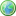Accessibility options:

# Search results

## Your search returned 57 resultsPolar form and De Moivre's Theorem - Numbas
3 questions. Finding modulus and argument of complex numbers. Use De Moivre's Theorem to find powers of complex numbersPolar Form and De Moivre's Theorem - Numbas
3 questions. Finding modulus and argument of complex numbers. Use De Moivre's Theorem to find powers of complex numbers. Numbas resources have been made available under a Creative Commons licence by the School of Mathematics & Statistics at Newcastle University.Systems of linear equations - Numbas
3 questions. First, two equations in two unknowns; second 3 equations in 3 unknowns, solved by Gauss elimination. The third, two equations in 2 unknowns solved by putting into matrix form and finding the inverse of the coefficient matrix. Numbas resources have been made available under a Creative Commons licence by Bill Foster and Christian Perfect, School of Mathematics & Statistics at Newcastle University.Second order differential equations - Numbas
5 questions on second order differential equations. First two and last, linear with constant coefficients; first two homogeneous, complex and repeated roots. Last, non-homogeneous. Third, motion under gravity. Fourth, linear with a given particular solution (variation of parameters). Numbas resources have been made available under a Creative Commons licence by the School of Mathematics & Statistics at Newcastle University.System of linear equations - Numbas
3 questions. First, two equations in two unknowns, second 3 equations in 3 unknowns, solved by Gauss elimination. The third two equations in 2 unknowns solved by putting into matrix form and finding the inverse of the coefficient matrix. Numbas resources have been made available under a Creative Commons licence by the School of Mathematics & Statistics at Newcastle University.Determinant of a matrix - Numbas
Find the determinants of three 2 x 2 invertible matrices.Logarithms and solving equations - Numbas
8 questions using logarithms. Of these 7 questions use logarithms to solve equations.

« Previous 1 2 3